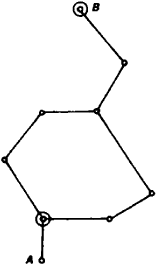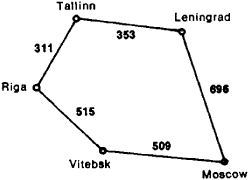# Graphs, Theory of

The following article is from The Great Soviet Encyclopedia (1979). It might be outdated or ideologically biased.

## Graphs, Theory of

a branch of finite mathematics characterized by a geometric approach to the study of objects. The basic concept of the theory is the graph, which is composed of a set of vertices (points) and a set of line segments (connections) linking some (possibly all) pairs of vertices. The pairs of vertices may also be connected by several line segments. Examples of graphs are a set of cities (the graph’s vertices) of, say, the Moscow Oblast and the roads connecting them (the graph’s line segments) or the elements of an electrical circuit and the wires connecting them. Figure 1 shows a graph in which the vertices are the stations of a cityFigure 1

subway system and the line segments connect the neighboring stations (one of the tasks is to indicate which route should be taken from station A to station B). A graph is oriented if its line segments are given an orientation, that is, if they indicate the order of the vertices. Finally, the theory of graphs studies graphs in which the line segments are given some weight or symbol as well as graphs in which special vertices, called poles, are distinguished. Examples include a diagram of the states of an automaton or a railroad network with the length of the lines or traffic capacity indicated on the arcs. Figure 2Figure 2

represents highways between Moscow and Tallinn. The problem is, for example, to choose the route of the least total distance from Moscow to Tallinn (these two cities are the poles of the network). A comparison of the two routes Moscow-Leningrad-Tallinn and Moscow-Vitebsk-Riga-Tallinn indicates that the route through Leningrad is shorter (1,049 km).

One of the first works on the theory of graphs was L. Euler’s (1736) work on the solution of puzzles and entertaining mathematical problems. The first important results were obtained in the first half of the 20th century in connection with the solution of problems encountered when constructing electrical circuits and computing chemical substances with various types of molecular bonds. However, the theory of graphs has developed extensively only since 1950’s. This has been the result of the establishment of cybernetics and the development of computer technology, which substantially enriched the theory of graphs with new material and new approaches and which also initiated the systematic study of graphs from various points of view (structural, informational, and others). It was precisely at this time that the problems and methods of the theory of graphs were formulated.

The theory of graphs is applied in the theory of programming; computer design; the study of physical, chemical, and technological processes; the solution of problems of planning; and linguistic and sociological investigations. The theory of graphs is closely related to classical mathematics as well as to new branches of mathematics; these include topology, algebra, combinatorial analysis, number theory, and the theory of the minimization of Boolean functions.

The theory of graphs comprises a large number of varied problems. Some of them are grouped in separate trends, while others are more isolated. Among the areas of the theory that have formed, one should mention problems relating to the analysis of graphs and the determination of various characteristics of their structure, for example, the clarification of the connectedness of a graph: can one go from one vertex to any other; calculating graphs or their parts having set properties, for example, calculating the number of trees with a given number of line segments (a tree is a nonoriented graph without cycles); and solving transportation problems connected with the transport of cargo along a network. There are solutions to a series of problems on the synthesis of graphs with given properties, for example, the construction of a graph with assigned degrees of vertices (the degree of a vertex is the number of line segments leading from it). There is an applied and theoretical significance to the problem of clarifying the possibility of the positioning of a graph on a surface without its line segments intersecting one another (that is, is a given graph planar). Another problem is that of dividing the graph into the minimum number of planar graphs. There are methods worked out for solving some problems of the theory of graphs (the aforementioned ones are by no means all). Some of these methods are Pólya’s method for the enumeration and calculation of graphs with assigned properties, the Ford-Fulkerson theorem and algorithm for solving transportation problems, and the “Hungarian” algorithm for solving assignment problems. Almost all problems in the theory of finite graphs (it is graphs with a finite number of vertices that are of practical interest) can be solved by sorting out a large number of choices (so-called complete sorting). For this reason they demand the construction of effective algorithms and the use of fast computers. Such problems include the coloration of the vertices of the graph, the determination of the identity of two graphs, and the traveling salesman problem. There are problems requiring an answer on principle, for example, the coloration of planar graphs and the reconstruction of a graph according to its subgraphs.

### REFERENCES

Berge, C. Teoriia grafov i ee primeneniia. Moscow, 1962. (Translated from French.)
Ore, O. Grafy i ikh primenenie. Moscow, 1965. (Translated from English.)
Zykov, A. A. Teoriia konechnykh grafov. Novosibirsk, 1969.
The Great Soviet Encyclopedia, 3rd Edition (1970-1979). © 2010 The Gale Group, Inc. All rights reserved.
Site: Follow: Share:
Open / Close